# 读取数据

``````\$xl=New-Object -ComObject "Excel.Application"
``````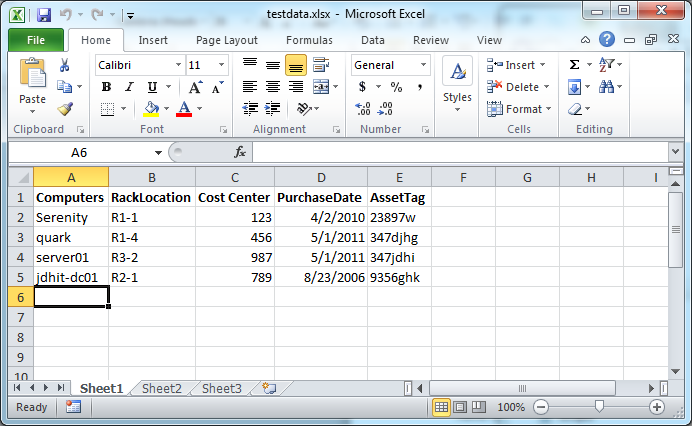``````\$wb=\$xl.Workbooks.Open(\$file)
\$ws=\$wb.ActiveSheet
``````

\$ws对象是我们对数据最重要的的引用点。我需要用的数据从A2单元格开始。在我的测试环境中，我也许知道我需要处理多少行，但是既然我知道从哪儿开始，我可以用一个Do循环来读取每一行，获取数据，进行进一步操作。

``````\$Row=2

do {
\$data=\$ws.Range("A\$Row").Text
...
``````

``````if (\$data) {
Write-Verbose "Querying \$data"
\$ping=Test-Connection -ComputerName \$data -Quiet
``````

``````if (\$Ping) {
\$OS=(Get-WmiObject -Class Win32_OperatingSystem -Property Caption -computer \$data).Caption
}
else {
\$OS=\$Null
``````

``````New-Object -TypeName PSObject -Property @{
Computername=\$Data.ToUpper()
OS=\$OS
Ping=\$Ping
Location=\$ws.Range("B\$Row").Text
AssetAge=((Get-Date)-(\$ws.Range("D\$Row").Text -as [datetime])).TotalDays -as [int]
}
``````

loop循环的最后一步是使行计数器自增1。

``````\$Row++
} While (\$data)
``````

``````\$xl.displayAlerts=\$False
\$wb.Close()
\$xl.Application.Quit()
``````

``````PS C:\scripts> .\Demo-ReadExcel.ps1

AssetAge     : 687
Ping         : True
Computername : SERENITY
Location     : R1-1
OS           : Microsoft Windows 7 Ultimate

AssetAge     : 293
Ping         : True
Computername : QUARK
Location     : R1-4
OS           : Microsoft Windows 7 Professional

AssetAge     : 293
Ping         : False
Computername : SERVER01
Location     : R3-2
OS           :

AssetAge     : 2005
Ping         : True
Computername : JDHIT-DC01
Location     : R2-1
OS           : Microsoft(R) Windows(R) Server 2003, Enterprise Edition
``````

# PowerShell 技能连载 - 在文件管理器中显示隐藏文件

PowerShell可以方便地读写注册表。注册表是Windows设置的中心仓库。

``````function Show-HiddenFile
{
param([Switch]\$Off)

\$value = -not \$Off.IsPresent
-Name Hidden -Value \$value -type DWORD

\$shell = New-Object -ComObject Shell.Application
\$shell.Windows() |
Where-Object { \$_.document.url -eq \$null } |
ForEach-Object { \$_.Refresh() }
}
``````

`Show-HiddenFile` 使得隐藏文件变得可见而 `Show-HiddenFile -Off` 使得隐藏文件不可见。操作结果几乎在所有文件管理窗口中立即生效。如果您在没有打开文件管理窗口的情况下做出改变，则改变不会立即生效，因为没有可以调用 `Refresh()` 方法的窗口。

# 创建一个简单的报表

Microsoft Excel包含一个非常复杂的COM对象模型，我们可以在Windows Powershell中和它交互。让我们从头开始创建一个Excel应用程序的对象。

``````PS C:\> \$xl=New-Object -ComObject "Excel.Application"
``````

``````PS C:\> get-process excel

Handles  NPM(K)    PM(K)      WS(K) VM(M)   CPU(s)     Id ProcessName
-------  ------    -----      ----- -----   ------     -- -----------
203      23    16392      24340   267     0.28   1280 EXCEL
``````

``````PS C:\> \$wb=\$xl.Workbooks.Add()
``````

``````PS C:\> \$ws=\$wb.ActiveSheet
``````

``````PS C:\> \$xl.Visible=\$True
``````

``````PS C:\> \$cells=\$ws.Cells
``````

``````PS C:\> \$cells.item(1,1)
``````

``````PS C:\> \$cells.item(1,1)=\$env:computername
``````

``````PS C:\> \$cells.item(1,2)=\$env:username
PS C:\> \$cells.item(2,1)=(get-Date)
``````

``````PS C:\> \$cells.item(1,1).font.bold=\$True
PS C:\> \$cells.item(1,2).font.bold=\$True
PS C:\> \$cells.item(1,1).font.size=16
PS C:\> \$cells.item(1,2).font.size=16
``````

``````PS C:\> \$wb.SaveAs("c:\work\test.xlsx")
``````

To fully exit, we’ll close the workbook and quit Excel.

``````PS C:\> \$wb.Close()
PS C:\> \$xl.Quit()
``````

``````Param([string]\$computer=\$env:computername)

#get disk data
\$disks=Get-WmiObject -Class Win32_LogicalDisk -ComputerName \$computer -Filter "DriveType=3"

\$xl=New-Object -ComObject "Excel.Application"

\$ws=\$wb.ActiveSheet

\$cells=\$ws.Cells

\$cells.item(1,1)="{0} Disk Drive Report" -f \$disks.SystemName
\$cells.item(1,1).font.bold=\$True
\$cells.item(1,1).font.size=18

#define some variables to control navigation
\$row=3
\$col=1

"Drive","SizeGB","FreespaceGB","UsedGB","%Free","%Used" | foreach {
\$cells.item(\$row,\$col)=\$_
\$cells.item(\$row,\$col).font.bold=\$True
\$col++
}

foreach (\$drive in \$disks) {
\$row++
\$col=1
\$cells.item(\$Row,\$col)=\$drive.DeviceID
\$col++
\$cells.item(\$Row,\$col)=\$drive.Size/1GB
\$cells.item(\$Row,\$col).NumberFormat="0"
\$col++
\$cells.item(\$Row,\$col)=\$drive.Freespace/1GB
\$cells.item(\$Row,\$col).NumberFormat="0.00"
\$col++
\$cells.item(\$Row,\$col)=(\$drive.Size - \$drive.Freespace)/1GB
\$cells.item(\$Row,\$col).NumberFormat="0.00"
\$col++
\$cells.item(\$Row,\$col)=(\$drive.Freespace/\$drive.size)
\$cells.item(\$Row,\$col).NumberFormat="0.00%"
\$col++
\$cells.item(\$Row,\$col)=(\$drive.Size - \$drive.Freespace) / \$drive.size
\$cells.item(\$Row,\$col).NumberFormat="0.00%"
}

\$xl.Visible=\$True

\$filepath=Read-Host "Enter a path and filename to save the file"

if (\$filepath) {
\$wb.SaveAs(\$filepath)
}
``````

``````\$cells.item(1,1)="{0} Disk Drive Report" -f \$disks.SystemName
\$cells.item(1,1).font.bold=\$True
\$cells.item(1,1).font.size=18
``````

``````\$row=3
\$col=1
``````

``````"Drive","SizeGB","FreespaceGB","UsedGB","%Free","%Used" | foreach {
\$cells.item(\$row,\$col)=\$_
\$cells.item(\$row,\$col).font.bold=\$True
\$col++
}
``````

``````foreach (\$drive in \$disks) {
\$row++
\$col=1
\$cells.item(\$Row,\$col)=\$drive.DeviceID
\$col++
\$cells.item(\$Row,\$col)=\$drive.Size/1GB
\$cells.item(\$Row,\$col).NumberFormat="0"
\$col++
...
``````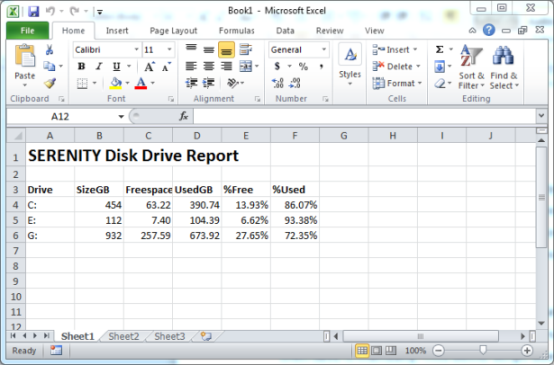# 创建一个富Excel文档

``````\$disks=Get-WmiObject -Class Win32_LogicalDisk -ComputerName \$computer -Filter "DriveType=3"
\$xl=New-Object -ComObject "Excel.Application"
``````

``````\$xlConditionValues=[Microsoft.Office.Interop.Excel.XLConditionValueTypes]
\$xlTheme=[Microsoft.Office.Interop.Excel.XLThemeColor]
\$xlChart=[Microsoft.Office.Interop.Excel.XLChartType]
\$xlIconSet=[Microsoft.Office.Interop.Excel.XLIconSet]
\$xlDirection=[Microsoft.Office.Interop.Excel.XLDirection]
``````

``````\$range=\$ws.range("A1")
\$range.Style="Title"
#或者用这种方法
``````

``````\$ws.columns.item("C:C").columnwidth=15
\$ws.columns.item("D:F").columnwidth=10.5
\$ws.columns.item("B:B").EntireColumn.AutoFit() | out-null
``````

``````\$start=\$ws.range("F4")
#获取最后一个单元格
\$Selection=\$ws.Range(\$start,\$start.End(\$xlDirection::xlDown))
#增加图标集
\$Selection.FormatConditions.item(\$(\$Selection.FormatConditions.Count)).SetFirstPriority()
\$Selection.FormatConditions.item(1).ReverseOrder = \$True
\$Selection.FormatConditions.item(1).ShowIconOnly = \$False
\$Selection.FormatConditions.item(1).IconSet = xlIconSet::xl3TrafficLights1
\$Selection.FormatConditions.item(1).IconCriteria.Item(2).Type = xlConditionValues::xlConditionValueNumber
\$Selection.FormatConditions.item(1).IconCriteria.Item(2).Value = 0.8
\$Selection.FormatConditions.item(1).IconCriteria.Item(2).Operator = 7
\$Selection.FormatConditions.item(1).IconCriteria.Item(3).Type = xlConditionValues::xlConditionValueNumber
\$Selection.FormatConditions.item(1).IconCriteria.Item(3).Value = 0.9
\$Selection.FormatConditions.item(1).IconCriteria.Item(3).Operator = 7
``````

（译者注：原文为I wish I could give you a set of translation rules, but it just takes trial and error and experience. Notice the use of the constant values?）

``````\$chart=\$ws.Shapes.AddChart().Chart
\$chart.chartType=\$xlChart::xlBarClustered
``````

``````\$start=\$ws.range("A3")
#获取最后一个单元格
\$Y=\$ws.Range(\$start,\$start.End(\$xlDirection::xlDown))
\$start=\$ws.range("F3")
#获取最后一个单元格
\$X=\$ws.Range(\$start,\$start.End(\$xlDirection::xlDown))
``````

``````\$chartdata=\$ws.Range("A\$(\$Y.item(1).Row):A\$(\$Y.item(\$Y.count).Row),F\$(\$X.item(1).Row):F\$(\$X.item(\$X.count).Row)")
\$chart.SetSourceData(\$chartdata)
``````

``````\$chart.seriesCollection(1).Select() | Out-Null
\$chart.SeriesCollection(1).ApplyDataLabels() | out-Null
\$chart.ChartTitle.Text = "Utilization"
``````

Excel很可能并不会按您所希望的位置摆放这个图表，所以您可以使用以下代码来定位它：

``````\$ws.shapes.item("Chart 1").top=40
\$ws.shapes.item("Chart 1").left=400
``````

`Top``Left` 是从Excel窗口开始计算的顶边距和左边距。可能会在获取右边距的时候遇到一些障碍和错误，但请注意在多台计算机上进行测试。最后一步是将工作表以计算机名来命名。

``````\$xl.worksheets.Item("Sheet1").name=\$name
``````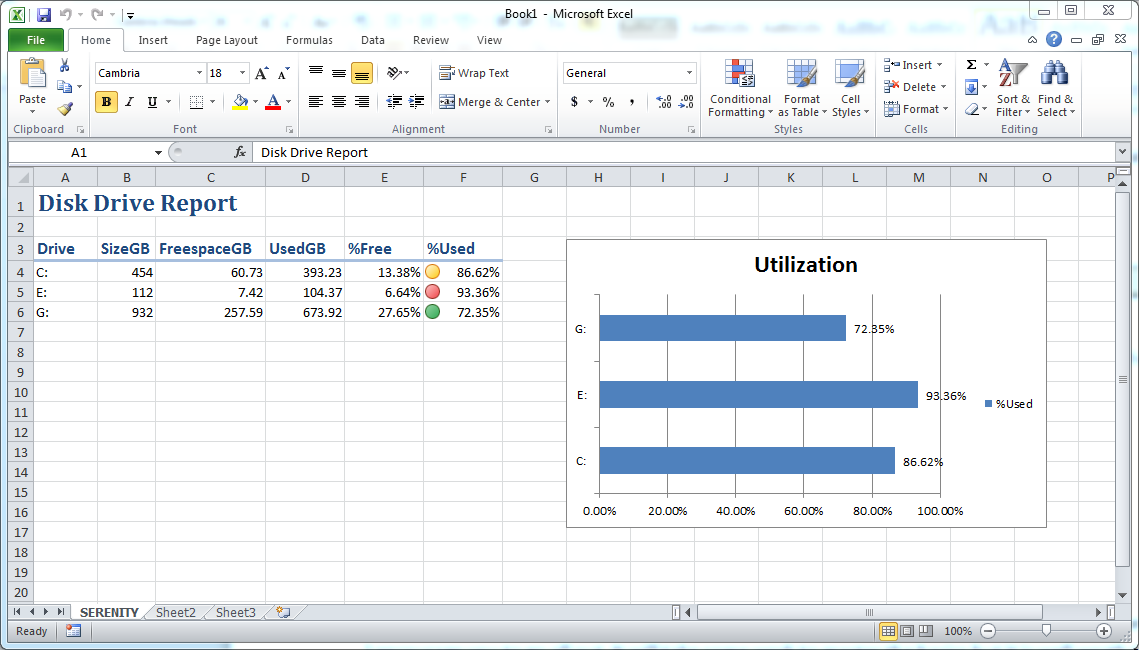# PowerShell 技能连载 - PowerShell函数的详细输出

（译者注：公共参数例如 `-Verbose``-Debug` 等）

``````function test
{
[CmdletBinding()]
param()
}
``````

``````function test
{
[CmdletBinding()]
param()

Write-Verbose "Starting"
"Doing Something"
Write-Verbose "Shutting Down"
}
``````

``````PS > test

Doing Something
``````

``````PS > test -Verbose

Starting
Doing Something
Shutting Down
``````

# PowerShell 技能连载 - 将结果复制到剪贴板

``````Get-Service | clip
``````

``````C:\>clip /?

CLIP

将命令行工具的输出重定向到 Windows 剪贴板。这个文本输出可以被粘贴
到其他程序中。

/?                  显示此帮助消息。

DIR | CLIP          将一份当前目录列表的副本放入 Windows 剪贴板。

``````

# PowerShell 技能连载 - 将Out-GridView改造为选择对话框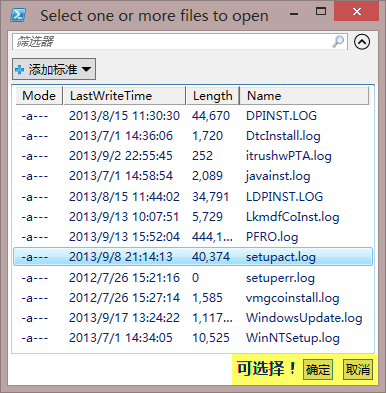``````\$Title = 'Select one or more files to open'

Get-ChildItem -Path \$env:windir -Filter *.log |
Out-GridView -PassThru -Title \$title |
ForEach-Object {
}
``````

# 理解PowerShell的类型转换魔法

PowerShell 的 `TimeSpan` 参数将10理解为10个计时周期，10:00理解为10小时，”10”理解为10天。

### 10. TypeConverter 转换

``````[TimeSpan] 10
[TimeSpan] "10"
[TimeSpan] "0:10"
``````

Lee Holmes [MSFT]
Windows PowerShell开发团队

# PowerShell 技能连载 - 3个最有用的ISE技巧

1. `CTRL+J` 打开一系列包含 `loops``conditions``functions` 以及 `workflows` 等内置代码片段的清单。这个技巧可以帮助您快速起步。

2. 当您选取一段代码后，按下 `F8` 将执行选中部分的代码。这个技巧在被注释的区域里也可以使用。所以您可以用 `<#``#>` 将一些代码注释掉，当需要执行的时候，选中它们并按下 `F8` 键。

3. 若要获得cmdlet的上下文帮助，点击脚本中的cmdlet并按 `F1` 键。

# PowerShell 技能连载 - 竖排的网格视图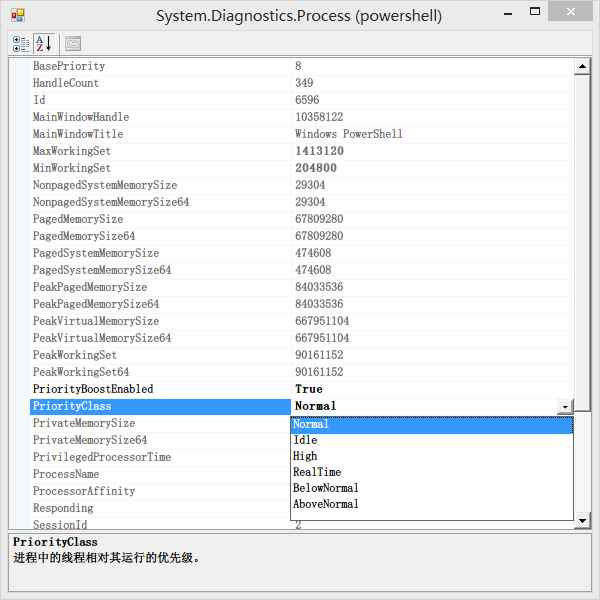``````Function Show-Object
{
param
(
[Parameter(Mandatory=\$true,ValueFromPipeline=\$true)]
[Object]
\$InputObject,

\$Title
)

if (!\$Title) { \$Title = "\$InputObject" }
\$Form = New-Object "System.Windows.Forms.Form"
\$Form.Size = New-Object System.Drawing.Size @(600,600)
\$PropertyGrid = New-Object System.Windows.Forms.PropertyGrid
\$PropertyGrid.Dock = [System.Windows.Forms.DockStyle]::Fill
\$Form.Text = \$Title
\$PropertyGrid.SelectedObject = \$InputObject
\$PropertyGrid.PropertySort = 'Alphabetical'
``````Get-Process -id \$pid | Show-Object